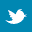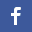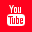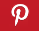Completely Solved C, C++ Programs Assignment.Quick Search Database Videos Tutorials Ebooks Forums FAQ Aboutus Household Industrial Manufacturing Service Shopping Transportation### C program to implement LAGRANGE'S INTERPOLATION METHOD.

 Filed Under: C Assignments

Program statement:
Write a C program to implement LAGRANGE'S INTERPOLATION METHOD.

Theory:
Interpolation means to find (approximate) values of a function y=f(x) for an x between different x-values x1, x2, …., xn+1 at which the values of f(x) are given.
So the given values y1=f(x1), y2=f(x2), …., yn=f(xn)
may come from a "mathematical function" given by a formula (which we may wish to approximate by a simple function) or from an "empirical function" resulting from observations or experiments.
A standard idea in interpolation now is to find a polynomial Pn(x) of degree n(or less)that assumes the given values ; thus
Pn(x1)=y1, Pn(x2)=y2, ……,Pn(xn)=yn
We call this Pn, an interpolation polynomial and x1, x2, …., xn the nodes. And if f(x) is a mathematical function, we call Pn an approximation of y(or a polynomial approximation).
We use Pn to get(approximate) values of f(x) between x1 and xn ("interpolation") or some-times outside the given interval ("extrapolation"). Also Pn satisfying (1) for given data exists and is unique.

Linear interpolation is interpolation by the straight line through (x1,y1), (x2y2). Fig.1 illustrates this. The same idea has been used in General Lagrange interpolation polynomial of degree n(or less).
Mathematical Formulation:
Here we are going to derive a formula for the polynomial of degree n which takes specific values at a given set of (n+1) points. Let , . . . . denotes (n+1) distinct Real number and let , , . . . . be the arbitrary real number. The points ( , ), ( , ), . . . ( , ) can be imagined to be data value connected by a curve. Any function y(x) satisfying the condition Y( )= , for k=1 to n+1 is called interpolation function. An interpolation is therefore a curve, which passes through the data points (( , ) , ( , ) . . . . .( , )).
We consider a second order polynomial of type
Y(x)= x- )(x- )+ (x- )(x- )+ (x- ) (x- ) . . . . . . (1)
Which passes through the points ( , ),( , ),( , ) where are unknown content whose value are to be determined.
(i) At x= y( )= x- )(x- )
=
(ii) At x= y( )= (x- )(x- )
=
(iii) At x= y( = (x- ) (x- )
=
Now, substituting the value of in the first equation we get
y(x)= + + . . . . . . (2)
y(x)=
It is a second order polynomial passing through 3 points. This polynomial is called Lagrange polynomial and is simple to program on a computer.
The Lagrange polynomial may be generalized to the n-th order as given below:
y(x)=
This formula is known as Lagrange interpolation formula.

ALGORITHM FOR IMPLEMENT OF LAGRANGIAN INTERPOLATION FORMULA:-
The following parameters are used in the below algorithm:
1. ‘n’ it indicates the no. of points on which the lagrangian interpolation formula will work.
2. ‘f(x)’ it indicates the given function corresponding to which, lagrangian interpolation formula will be implemented.
3. ‘x[i]’ it is a 1D array of size n, containing the corresponding values of x(from x to x[n]).
4. ‘y[i]’ it is also a 1D array of size n, containing the corresponding values of y(from y to y[n]).
5. ‘x’ it is a value of x where the interpolation function will be calculated.

The steps of algorithm written below:
step1: Two 1D array x[n], y[n] containing the values of x (from values of the function i.e the values of y(from y to y[n]). For a set of n points are declared.
step2: Write “ Enter the number of points:”
step4: If n<1 then goto step2
step5: Write “Enter the value of x”
step7: i=1
step8: Write “ Enter the value of x and y at the point:” , i
step10: i=i+1
step11: if i<=n then goto step8
step12: S=0
step13: i=1
step14: p=1
step15: j=1
step16: if (j!=i )then p=p*((x-x[i])/(x[i]-x[i]))
step17: j=j+1
step18: if j<=n then goto step16
step19: s=s+y[i]*p
step20: i=i+1
step21: if i<=n then goto step14
step22: Write ” The interpolation value is”, s
step23: End.

Program listing:
#include<stdio.h>
#include<math.h>
#define MAX 200
/**************************************************************/
/* Lagranges interpolation formula */
/*
Y=(x-X2)(x-X3)...(x-Xn)/(X1-X2)(X1-X3)...(X1-Xn)*y
+(x-X1)(x-X3)...(x-Xn)/(X2-X2)(X2-X3)...(X2-Xn)*y
+...+
(x-X1)(x-X2)...(x-X(n-1))/(Xn-X1)(Xn-X2)...(Xn-X(n-1))*y[n]
*/
/*************************************************************/
void main()
{
int n,i,j ;
char ch;
double x[MAX],f[MAX],fp,lf,sum,xp ;
clrscr();
printf("n--------------------------------------------------------------------------");
printf("nn LAGRANGE'S INTERPOLATION METHOD ");
printf("nn------------------------------------------------------------------------");
do
{
while((n<=0) || (n>200))
{
printf("n ENTER THE TOTAL NUMBER OF POINT FOR INTERPOLATION: ");
scanf("%d",&n);
if (n<=0)
printf("n NUMBER OF POINT FOR INTERPOLATION MUST BE POSITIVE!!!!!!n");
if (n>200)
printf("n THIS PROGRAM IS NOT CAPABLE TO ACCEPT THIS VALUE!!!!!!n");
}
printf("nn ENTER THE VALUE OF x & THE CORRESPONDING VALUES OF f(x)");
for(i=1;i<=n;i++)
{

printf("nn ENTER THE VALUE OF x%d= ",i);
scanf("%lf",&x[i]);
printf("n ENTER THE VALUE OF f(x%d)= ",i);
scanf("%lf",&f[i]);
}
printf("nn ENTER THE VALUE OF x AT WHICH INTERPOLATION IS REQUIRED: ");
scanf("%lf",&xp);
sum=0.0;
for(i=1;i<=n;i++)
{
lf=1.0;
for(j=1;j<=n;j++)
{
if(i!=j)
lf=lf*(xp-x[j])/(x[i]-x[j]);
}
sum=sum+lf*f[i];
}
fp=sum;
printf("n THE REQUIRED INTERPOLATION VALUE AT x=%lf IS: %lf",xp,fp);
printf("nn--------------------------------------------------------------");
printf("nDo you wish to continue[y/n]: ");
ch=(char)getche();
}while(ch=='Y' || ch=='y');
getch();
}

Output:
-----------------------------------------------------------------------------------------------------
LAGRANGES INTERPOLATION
-----------------------------------------------------------------------------------------------------
ENTER THE TOTAL NUMBER OF POINT FOR INTERPOLATION: 4
ENTER THE VALUE OF x & THE CORRESPONDING VALUES OF f(x)
ENTER THE VALUE OF x1= 1
ENTER THE VALUE OF f(x1)= 1
ENTER THE VALUE OF x2= 2
ENTER THE VALUE OF f(x2)= 8
ENTER THE VALUE OF x3= 3
ENTER THE VALUE OF f(x3)= 27
ENTER THE VALUE OF x4= 4
ENTER THE VALUE OF f(x4)= 64
ENTER THE VALUE OF x AT WHICH INTERPOLATION IS REQUIRED: 3.5
THE REQUIRED INTERPOLATION VALUE AT x=3.500000 IS: 42.875000
-----------------------------------------------------------------------------------------------------
Do you wish to continue[y/n]: n

Discussions :
● Consider the time complexity of Lagrange's interpolation. Paying attention the multiplication operator and ignoring the subtracting factor, we note that the time for each execution of the multiplication operator is proportional to the degree of P, which is less than or equal to j - 1 at the jth iteration. The total cost of the polynomial multiplication step is

This time complexity is discouraging, because its order is so high.
● Lagrange's Interpolation is simple to program on a computer. It is, however, extremely cumbersome to use for hand calculation. Further, there is no indication of the error committed in using this approximation and thus it is difficult to pick the order of the polynomial to be fitted. In the order of the polynomial does not necessarily yield a better accuracy of interpolation.
● In the Lagrange's Interpolation formula one of the main advantage is that there is no restriction on spacing and order of the tabulating points x0,x1,x2,…etc. However it has the disadvantage that if we want to increase the degree of interpolating polynomial by one more tabular point, the computations are to be made fresh ; the previous computations are of little help. This disadvantage is not present in Newton’s divided difference Interpolation formula.Back to main directory:Software Practical